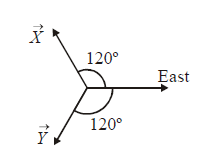# Complex Numbers Set 7

Go back to  'SOLVED EXAMPLES'

Example-  25

If \begin{align}\theta = \frac{{8\pi }}{{11}},\end{align}  find the value of $$\cos \theta + \cos 2\theta + \cos 3\theta + \cos 4\theta + \cos 5\theta$$ .

Solution: Consider the eleventh roots of unity, i.e., the solutions to the equation

${z^{11}} - 1 = 0$

The roots are $${e^{i2\,k\,\pi /11}},\,\,k = 0,\,\,1...10$$  .

The roots can also be expressed in terms of $${e^{i8k\pi /11}}$$  , i. e., if we let $$\alpha = {e^{i8k\pi /11}}$$  and take 11 consecutive values of k, we will still be able to list down all the roots (Think about why this should happen carefully. Take $$\alpha = {e^{i2k\pi /11}}$$ and take 11 consecutive values of k; and then take $$\alpha = {e^{i8k\pi /11}}$$  and again take 11 consecutive values of k. You will get the same set of 11 (eleventh roots of unity) values).

Thus, we let $$\alpha = {e^{i8k\pi /11}}$$ and let k take the values –5, –4, ... 4, 5 (eleven consecutive values)

\begin{align}& \Rightarrow \,\,\,\sum\limits_{k = - 5}^5 {{e^{i8\,k/11}} = 0} \\&\Rightarrow \,\,\,\sum\limits_{k = - 5 \atop k \ne 0 } ^5 {{e^{i8\,k/11}} = - 1}\\\\&\Rightarrow \,\,\,2(\cos \theta + \cos 2\theta + \cos 3\theta + \cos 4\theta + \cos 5\theta ) = - 1\\∵\left( {\,{e^{i\phi }} + {e^{ - i\phi }} = 2\cos \phi } \right)\\&\Rightarrow \,\,\,{\rm{The\; required \;sum \;is\;}} - \frac{1}{2}\end{align}

Example-  26

From the origin, a person moves 1 unit east, then \begin{align}\frac{1}{2}\end{align}  unit parallel to $$\vec X$$ , then \begin{align}\frac{1}{{{2^2}}}\end{align} unit parallel to $$\vec Y$$ , then \begin{align}\frac{1}{{{2^3}}}\end{align} unit east again, and so on.  After an infinite amount of time, find his distance from the origin.Solution: Observe that if the east direction is taken to be the positive real axis, then $$\vec X\;{\rm{and}}\;\vec Y$$  will be parallel to $$\omega \;{\rm{and}}\;{\omega ^2}$$. Thus, the motion of the person can be expressed as the following GP:

$1 + \frac{\omega }{2} + {\left( {\frac{\omega }{2}} \right)^2} + {\left( {\frac{\omega }{2}} \right)^3} + ...\infty = \frac{1}{{1 - \frac{\omega }{2}}} = \frac{2}{{2 - \omega }}$

Thus, the distance of the person from the origin after an infinite amount of time is

${d_\infty } = \left| {\;\frac{2}{{2 - \omega }}\;} \right|\; = \;\frac{2}{{\sqrt 7 }}$

Example-  27

The radius of a circle which circumscribes a regular n-sided polygon with vertices $${A_1},\;{A_2},\;....,{A_n}$$  is R.

(a)  Find the sum of the squares of all the sides and all the diagonals.

(b)  Find the sum of all the sides and all the diagonals.

(c)  Find the product of all the sides and all the diagonals.

Solution: Assume that the vertices are located at $${z_{k + 1}} = {{\mathop{\rm Re}\nolimits} ^{i\frac{{2\pi k}}{n}}}$$ , for $$k = 0,\;1,\;2,...,\;n - 1$$ . Note that $${z_1} = R$$ .

(a) Fix one vertex, say A1. Let us first calculate the sum

\begin{align}&{S_1} = {\left| {{A_1}{A_2}} \right|^2} + {\left| {{A_1}{A_3}} \right|^2} + ...{\left| {{A_1}{A_n}} \right|^2}\\ &\quad= \sum\limits_{j = 2}^n {{{\left| {{z_1} - {z_j}} \right|}^2}} = \sum\limits_{j = 2}^n {\left( {{{\left| {{z_1}} \right|}^2} + {{\left| {{z_j}} \right|}^2} - 2\left| {{z_1}} \right|\;\left| {{z_j}} \right|\cos \left( {{\theta _1} - {\theta _j}} \right)} \right)} \\ &\quad= (n - 1){R^2}\; + (n - 1){R^2}\; - 2{R^2}\,\sum\limits_{j = 2}^n {\cos \left( {{\theta _1} - {\theta _j}} \right)} \\ &\quad= (2n - 2){R^2} + 2{R^2} = 2n{R^2}\end{align}

The total sum S can be obtained by multiplying S1 by \begin{align}\frac{n}{2}\end{align} , that is, $$S = {n^2}{R^2}$$

(b) Once again, we fix one vertex, say A1, and calculate the sum

\begin{align}&{S_1} = \left| {{A_1}\;{A_2}} \right| + \left| {{A_1}\;{A_3}} \right| + ... + \left| {{A_1}\;{A_n}} \right|\\ &\quad= 2R\left( {\sin \frac{\pi }{n} + \sin \frac{{2\pi }}{n} + ... + \sin \frac{{(n - 1)\pi }}{n}} \right)\\ &\quad= 2R\cot \frac{\pi }{{2n}}\end{align}

The total sum \begin{align}S = \frac{n}{2}{S_1} = nR\cot \frac{\pi }{{2n}}\end{align}

(c) Once again, we calculate

\begin{align}&{P_1} = \left| {{A_1}\;{A_2}} \right| \times \left| {{A_1}\;{A_3}} \right| \times ...\left| {{A_1}\;{A_n}} \right|\\\\&{P_1} = \left| {\left( {{z_1} - {z_2}} \right)\;\left( {{z_1} - {z_3}} \right)...\left( {{z_1} - {z_n}} \right)} \right|\\\\ &\;\quad= \mathop {\lim }\limits_{z \to R} \;\left| {\frac{{{z^n} - {R^n}}}{{z - R}}} \right| = n{R^{n - 1}}\end{align}

The required product is

$P = P_1^{\frac{n}{2}} = {n^{\frac{n}{2}}}\;{R^{\frac{{n(n - 1)}}{2}}}$

Example-  28

If the points representing complex numbers $${z_1},{z_2},....,{z_n}$$  in the Argand plane lie on one side of a line drawn through the origin, then prove that the same is true for the points representing complex numbers \begin{align}\frac{1}{{{z_1}}},\frac{1}{{{z_2}}},....\frac{1}{{{z_n}}}.\end{align}

Also, show that \begin{align}{z_1} + {z_2} + ... + {z_n} \ne 0{\rm{ and }}\frac{1}{{{z_1}}} + \frac{1}{{{z_2}}} + ...\frac{1}{{{z_n}}} \ne 0.\end{align}

Solution: Recall that any line passing through the origin will have an equation of the form $$az + \bar a\;\bar z = 0$$. Assume that all $${z_i}$$ lie in the half-plane for which $$a{z_i} + \bar a\,{\bar z_i} > 0$$. Dividing both sides by \begin{align}{\left| {{z_i}} \right|^2} = {z_i}{\bar z_i}\end{align} , we have

\begin{align}\frac{a}{{{{\bar z}_i}}} + \frac{{\bar a}}{{{z_i}}} > 0\end{align}  for all i

Thus, all the points \begin{align}\frac{1}{{{z_i}}}\end{align} lie on the same side of the line $$\bar az + a\bar z = 0$$

Now, $$a{z_i} + \bar a\,\bar z { _i} > 0 \;\;\; \Rightarrow a\,\sum {{z_i} + \bar a\,\sum {{{\bar z}_i}} > 0}$$ . This tells us that $$\sum {{z_i}}$$ cannot be 0. Similarly, we can show that \begin{align}\sum {\frac{1}{{{z_i}}}}\end{align}  is also non-zero.

Learn from the best math teachers and top your exams

• Live one on one classroom and doubt clearing
• Practice worksheets in and after class for conceptual clarity
• Personalized curriculum to keep up with school